# Ex 13.5 NCERT Solutions- Surface Areas and Volumes Class 9 Notes | EduRev

## Class 9 : Ex 13.5 NCERT Solutions- Surface Areas and Volumes Class 9 Notes | EduRev

The document Ex 13.5 NCERT Solutions- Surface Areas and Volumes Class 9 Notes | EduRev is a part of the Class 9 Course Class 9 Mathematics by VP Classes.
All you need of Class 9 at this link: Class 9

Question 1. A matchbox measures 4 cm x 2.5 cm. x 1.5 cm. What will be the volume of a packet containing 12 such boxes?
Solution:
Measures of matchbox (cuboid) is 4 cm x 2.5 cm x 1.5 cm
⇒ l = 4 cm, b = 2.5 cm and h = 1.5 cm
∴ Volume of matchbox = (l x b) x h = [4 cm x 2.5 cm] x 1.5 cm3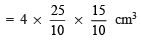= 15 cm
⇒ Volume of 12 boxes = 12 x 15 cm3 = 180 cm3

Question 2. A cuboidal water tank is 6 m long, 5 m wide and 4.5 m deep. How many litres of water can it hold? (1 m3 = 1000 l)
Solution:
Here, Length (l)= 6 m
Depth (h) = 4.5 m
∴ Capacity = l x b x h = 6 x 5 x 4.5 m
= 6 x 5 x (45/10) m= 3 x 45 = 135 m3

∵ 1 m3 can hold 1000 l.
∴ 135 m3 can hold (135 x 1000 l = 135000 l) of water.
∴ The required amount of water in the tank = 135000 l.

Question 3. A cuboidal vessel is 10 m long and 8 m wide. How high must it be made to hold 380 cubic metres of liquid?
Solution:
Length (l) = 10 m
Volume (v) = 380 m
Let height of the cuboid be ‘h’.
Since, volume of a cuboid = l x b x h
∴ Volume of the cuboidal vessel = 10 x 8 x h m3 = 80h m3
⇒ 80h = 380

h= 380/80 = 19/4 m = 4.75 m

Thus, the required height of the liquid = 4.75 m

Question 4. Find the cost of digging a cuboidal pit 8 m long, 6 m broad and 3 m deep at the rate of  30 per m3.
Solution:
Length (l)= 8 m
Depth (h) = 3 m
∴ Volume of the cuboidal pit = l x b x h = 8 x 6 x 3 m3
= 144 m
Since, rate of digging the pit is ₹ 30 per m3.
∴ Cost of digging = ₹ 30 x 144 = ₹ 4320

Question 5. The capacity of cuboidal tank is 50000 litres of water. Find the breadth of the tank, if its length and depth are respectively 2.5 m and 10 m.
Solution:
Length of the tank (l) = 2.5 m
Depth of the tank (h) = 10 m
Let breadth of the tank = b m
∴ Volume (capacity) of the tank = l x b x h = 2.5 x b x 10 m3 = (25/10) x 10 x b m= 25b m3
But the capacity of the tank = 50000 l = 50 m3                   [∵ 1000 l = 1 m3]
∴ 25b = 50 m3
⇒ b= (50/25) m = 2 m
Thus, the depth of the tank = 2 m

Question 6. A village, having a population of 4000, requires 150 litres of water per head per day.
It has a tank measuring 20 m x 15 m x 6 m. For how many days will the water of this tank last?
Solution:
Length of the tank (l) = 20 m
Breadth of the tank (b) = 15 m
Height of the tank (h) = 6 m
∴ Volume of the tank = l x b x h = 20 x 15 x 6 m3 = 1800 m
Since 1 m3 = 1000 l
∴ Capacity of the tank = 1800 x 1000 l = 1800000 l
Village population = 4000

Since, 150 l of water is required per head per day.
∴ Amount of water is required per day = 150 x 4000 l.
Let the required number of days = x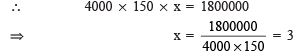Thus, the required number of days is 3.

Question 7. A godown measures 60 m x 25 m x 10 m. Find the maximum number of wooden crates each measuring 1.5 m x 1.25 m x 0.5 m that can be stored in the godown.
Solution:

volume of the godown = 60x 25 x 10 m3

Volume of a crate = 1.5 x 1.25 x 0.5 m3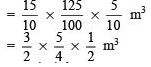Let the required number of crates is ‘n’.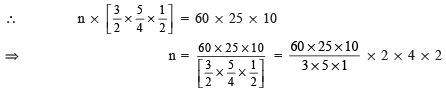= 16000

Question 8. A solid cube of side 12 cm is cut into eight cubes of equal volume. What will be the side of the new cube? Also, find the ratio between their surface areas.
Solution:
Side of the given cube = 12 cm
∴ Volume of the given cube = (side)3 = (12)3 cm
Side of the smaller cube:
Let the side of the new (smaller) cube = n
⇒ Volume of smaller cube = n
⇒ Volume of 8 smaller cubes = 8n3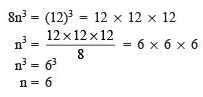Thus, the required side of the new (smaller) cube is 6 cm.
Ratio between surface areas:
Surface area of the given cube = 6 x (side)2 = 6 x 122 cm
= 6 x 12 x 12 cm2
Surface area of one smaller cube = 6 x (side)= 6 x 62 cm
= 6 x 6 x 6 cm2
∴ Surface area of 8 smaller cubes = 8 x 6 x 6 x 6 cm2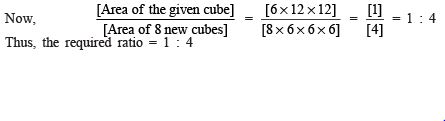Question 9. A river 3 m deep and 40 m wide is flowing at the rate of 2 km per hour. How much water will fall into the sea in a minute?
Solution:
The water flowing in a river can be considered in the form of a cuboid.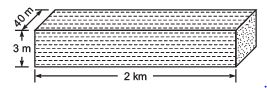Such that Length (l) = 2 km = 2000 m
Depth (h) = 3 m
∴ Water volume (volume of the cuboid so formed) = l x b x h = 2000 x 40 x 3 m3
Now, volume of water fallowing in 1 hr (= 60 minutes) = 2000 x 40 x 3 m3
∴ Volume of water that will fall in 1 minute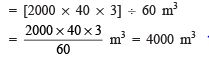VOLUME OF A CYLINDER

For a right circular cylinder, if ‘r’ be the base radius and ‘h’ be its height then,
Volume = Base area x height
= πr 2h

Offer running on EduRev: Apply code STAYHOME200 to get INR 200 off on our premium plan EduRev Infinity!

132 docs

,

,

,

,

,

,

,

,

,

,

,

,

,

,

,

,

,

,

,

,

,

;